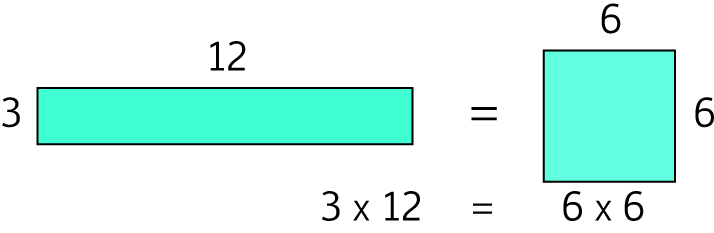# Definition of Geometric Mean

The geometric mean of $n$ numbers is equal to the $n$th root of the product of the numbers. It is a special type of average.

The geometric mean of two numbers is the square root of their product, the geometric mean of three numbers is the cube root of their product, and so on.

For example, the geometric mean of $3$ and $12$ is equal to $\sqrt{(3 \times 12)} = \sqrt{36} = 6$.### Description

The aim of this dictionary is to provide definitions to common mathematical terms. Students learn a new math skill every week at school, sometimes just before they start a new skill, if they want to look at what a specific term means, this is where this dictionary will become handy and a go-to guide for a student.

### Audience

Year 1 to Year 12 students

### Learning Objectives

Learn common math terms starting with letter G

Author: Subject Coach
You must be logged in as Student to ask a Question.